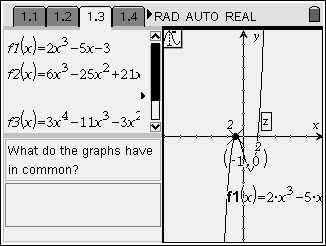# Activities

••• ##### Subject Area

• Math: Precalculus: Complex Numbers
• Math: Precalculus: Polynomial, Power, and Rational Functions

• ##### Author9-12

15 Minutes

• ##### Device
• TI-Nspire™
• TI-Nspire™ CAS
• ##### Software

TI-Nspire™
TI-Nspire™ CAS

1.7

## It's a Rat Race for the Zeros#### Activity Overview

In this introductory activity, students will use a program and graphing to help them explore the Rational Root Theorem. They will compare sets of functions that have at least one real zero or have no real roots and then determine how the factors of the first coefficient and the constant can produce a zero.

#### Key Steps

•On pages 1.2 and 1.3, students are given 2 sets of functions. They are to graph the functions one at a time and find the zeros using the Graph Trace feature (MENU > Trace > Graph Trace). When students reach a zero a z will appear. If the y-value of the coordinate is 0, then the x-value is rational. If the y-value of the coordinate is not 0, then the x-value is irrational.